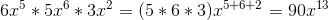# ISEE Upper Level Math : How to multiply exponents

## Example Questions

2 Next →

### Example Question #21 : Exponents

What is the value of this equation?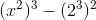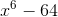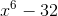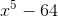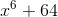Explanation:

When an exponent is raised to another exponent, the exponents should be multiplied toghether. This will result in: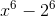### Example Question #11 : How To Multiply Exponents

Which expression is equal to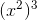?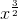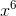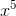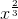Explanation:

When exponent of a value is raised to another exponent, the values of the exponents are multiplied by each other.2 is multiplied by 3, and so the exponent of x is 6.### Example Question #21 : Exponents

What is the value of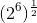?Explanation:

When one exponent is raised to another exponent, the values of the exponents should be multiplied together. Thus,can be simplified to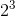, given that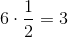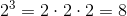### Example Question #72 : Numbers And Operations

What is the expression below equal to?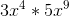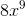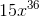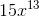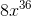Explanation:

When exponents are multiplied by each other, the powers should be added together. Meanwhile, numbers not raised to an exponent are simply multiplied by each other.

Therefore, the answer is, because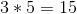, and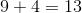### Example Question #23 : Exponents

What is the value of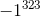?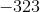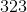Explanation:

1 raised to any exponent will always be 1.

-1 will be equal to 1 when the exponent is even and will be equal to -1 when the exponent is odd.

Given that 323 is odd,is equal to -1.

### Example Question #21 : Exponents

Simplify the following: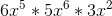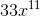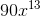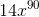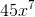Explanation:

Simplify the following:Let's begin by recalling two rules

1) When multiplying variables with a common base, add the exponents.

2) When multiplying variables with a common base, multiply the coefficients.